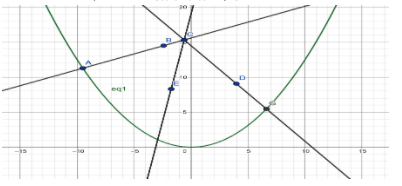Courses
Courses for Kids
Free study material
Free LIVE classes
More# Find the locus of the point of intersection of those normal to the parabola ${{x}^{2}}=8y$ which are at right angles to each other.Verified
327.3k+ views
Hint- Apply the concept of co-normal points over here. Co normal points are the feet of any three normal that are drawn from any point. Note that, there can be at the most, only 3 normals possible to be drawn on a parabola from a single point. You’ll eventually get a cubic equation in their slopes, and apply general results of cubic equations, like sum of roots, sum of product of roots taken two at a time, and the product of the roots, to get to the final answer.

Let’s assume a parabola ${{x}^{2}}=8y$.General equation of normal of the parabola ${{x}^{2}}=8y$is
$x=ym-2bm-b{{m}^{3}}$ …………………. (1)
Where $\dfrac{1}{m}=$slope of normal
As we can see that the equation of normal $x=ym-2bm-b{{m}^{3}}$is a $3$ degree polynomial in $m$.
Therefore; this equation will have three solutions.
It means three normals can be drawn on a parabola from one point, lying anywhere.
Let the point of the intersection of normal is $C\left( h,k \right)$.
Therefore, point $C$ will satisfy the equation (1).
From equation (1) and the point $C$, we get :
$h=km-2bm-b{{m}^{3}}$
$\Rightarrow b{{m}^{3}}+m\left( 2b-k \right)+h=0$ ……….. (2)
Let ${{m}_{1}},{{m}_{2}}\,and\,{{m}_{3}}$are solutions of equation (2).
Therefore,
${{m}_{1}}+{{m}_{2}}+{{m}_{3}}=0$,
${{m}_{1}}{{m}_{2}}+{{m}_{2}}{{m}_{3}}+{{m}_{3}}{{m}_{1}}=\dfrac{\left( 2b-k \right)}{b}$ and
${{m}_{1}}{{m}_{2}}{{m}_{3}}=-\dfrac{h}{b}$ …….. (3)
Let’s assume ${{m}_{1}}\,and\,{{m}_{2}}\,$are the slopes of two normals which intersect normally each other at point $C$.
Therefore, the product of their slopes $=-1$
${{m}_{1}}{{m}_{2}}=-1$ ………. (4)
Put the value of ${{m}_{1}}{{m}_{2}}=-1$in equation (3)
Therefore, from equation (3) and (4), we get :
$\left( -1 \right){{m}_{3}}=-\dfrac{h}{b}$
$\Rightarrow {{m}_{3}}=\dfrac{h}{b}$ ……… (5)
As ${{m}_{3}}$is also a solution to equation (2), it will satisfy the equation.
Therefore, from equation (2) and (5)
Put the value of ${{m}_{3}}=\dfrac{h}{b}$ in equation (3), we get :
$\Rightarrow b{{\left( \dfrac{h}{b} \right)}^{3}}+\dfrac{h}{b}\left( 2b-k \right)+h=0$
$\Rightarrow {{\dfrac{h}{{{b}^{2}}}}^{3}}+2h-\dfrac{hk}{b}+h=0$
Taking $h$ common, we get :
$\Rightarrow h\left( \dfrac{{{h}^{2}}}{{{b}^{2}}}+3-\dfrac{k}{b} \right)=0$
$\therefore \dfrac{{{h}^{2}}}{{{b}^{2}}}+3-\dfrac{k}{b}=0$
$\Rightarrow {{h}^{2}}+3{{b}^{2}}-kb=0$
$\Rightarrow {{h}^{2}}=b\left( k-3b \right)$ ……….. (6)
Interchange $\left( h,k \right)\to \left( x,y \right)$and equation (6) becomes
$\Rightarrow {{x}^{2}}=b\left( y-3b \right)$ …………. (7)
According to the question, the given parabola is ${{x}^{2}}=8y$.
Comparing with the general equation of a parabola ${{x}^{2}}=4ay$
${{x}^{2}}=4by$ ……… (A)
${{x}^{2}}=8y$ ………… (B)
From (A) and (B),
$b=2$ Put this value in equation (7)
Form equation (7)
$\Rightarrow {{x}^{2}}=2\left( y-3\times 2 \right)$
$\Rightarrow {{x}^{2}}=2\left( y-6 \right)$ Locus of point of intersection.

Note: We can also start from parabola ${{y}^{2}}=4ax$and there general equation of normal$y=xm-2am-a{{m}^{3}}$. But at the end of calculation just interchange the values
$x\to y,y\to x\,and\,a\to b$.
Last updated date: 01st Jun 2023
Total views: 327.3k
Views today: 8.85k